# Understanding Kinematics and Newton’s Laws of Motion

March 6, 2013 —Comments Off

Throughout the history of physics, scientists have postulated laws and theories about the nature of the world around them. Some were proven false, while others have grown to be the basis of entire fields of study. One such field is classical mechanics, which describes the area of physics most familiar to us, that of the motion of macroscopic objects, from baseballs to planets and traveling along hills to falling from space. As one of the oldest subjects in science, the work here serves as a basis for less familiar areas such as relativity and quantum mechanics.

A crucial set of laws for classical mechanics are those proposed by Isaac Newton. “Newton’s laws of motion” underlie all of our theories about the motion of particles. His first law of motion simply states that an object that experiences no net force will continue to move at a constant velocity, which means that if it is not moving it will remain stationary, and if it is moving it will continue to move at the same rate. Thus if something is moving at “20 mph for 30 minutes” and no outside forces act on it, it will have moved 10 miles.

Newton’s second law is more interesting. It tells us a force acting on an object will accelerate that object in the same direction as the force and inversely proportionally to its mass. This gives us the familiar equation: F = m × a

Acceleration is simply the rate at which the velocity of an object changes with time. From the definition of acceleration, we can construct much of kinematics. We can determine how far an object with an acceleration of “2 meters per second squared is after 10 minutes”. We can find acceleration by working backward, such as the case of an object “moving 2 miles in 100 seconds with initial velocity 5 meters per second”. From these calculations and Newton’s first two laws, we can derive a host of other equations.

Let’s look at a very common form of acceleration, one that we are all exposed to, “gravity”. As many of us know our “theory of gravity” also starts with Isaac Newton. Newton worked out that the force between two objects due to gravity was proportional to their mass and and inversely proportional to their distance. This force, however, is quite small. The “gravitational force between 100 kg and 200 kg at 10 meters” is only 1.335 × 10-8 newtons. Only with objects on the scale of a planet (“Earth’s mass” is 5.9721986 × 1024 kilograms) can we get the level of gravity we feel on Earth.

Returning to Newton’s second law of motion, let’s see how gravity accelerates objects here on Earth. Within a few miles of the Earth’s surface, the “acceleration of gravity” is fairly constant. Wolfram|Alpha can tell you how the strength of gravity diminishes above your location, for example at 4 miles up. Even at 4 miles, the acceleration due to gravity is within 1% of its ground level value.

With a relatively constant acceleration at low altitude, we can look at how long it takes to fall from a given height. Essentially, we take an object at rest and allow the force of gravity to move it. For a concrete example, we will find the “time to fall from the Empire State Building”.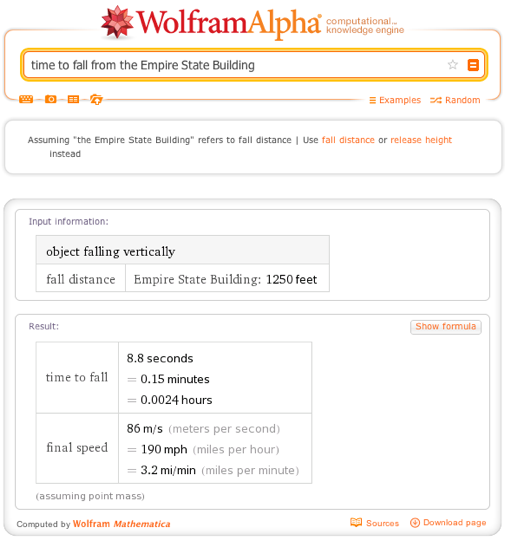Of course this calculation is for an idealized world without air resistance. The force of the air hitting our falling object will counteract the acceleration due to gravity. Asking Wolfram|Alpha for the “time to fall from the Empire State Building with drag” adds in this extra factor. Assuming our falling object is a box, we will need to adjust the drag coefficient to 0.8 (a falling cube varies in its drag coefficient from 0.8 to 1.05 depending on its facing). From this we find that a box falling from the Empire State Building will reach terminal velocity, the speed where the force of gravity pulling the object down is completely equaled by the force of air resistance pushing it up. At this point the net force is zero and by Newton’s first law of motion, the box moves at a constant speed—until another force acts on the object, like the ground.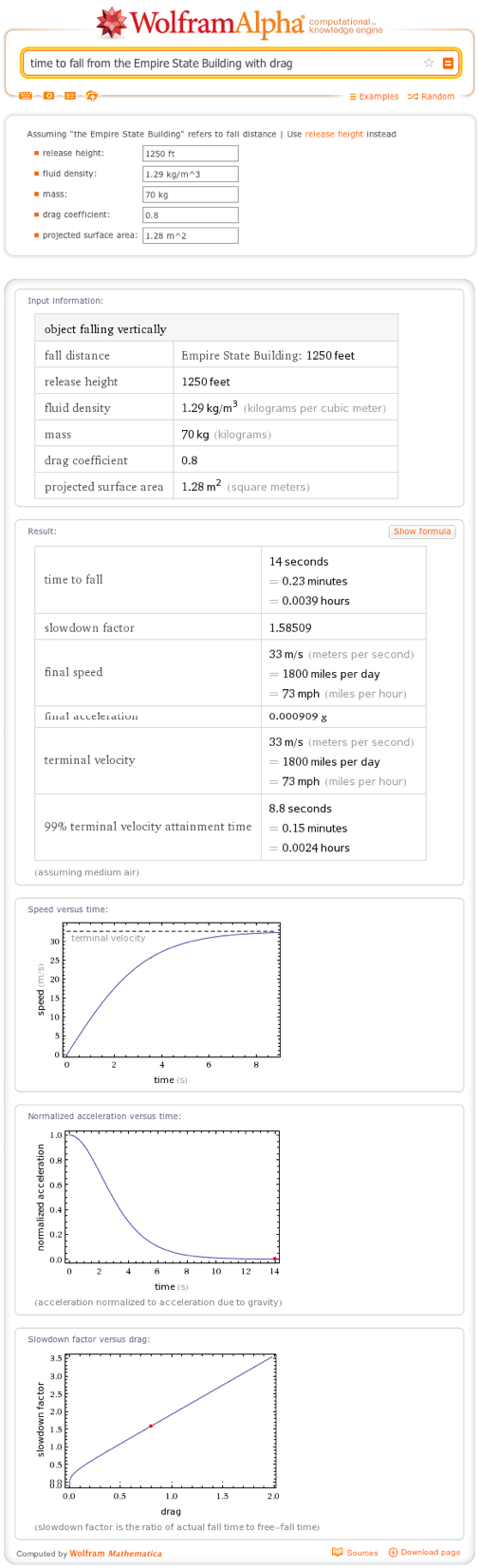Next we will look at Newton’s first and second laws together in two-dimensional motion. Specifically, let’s look at projectile motion, for example a superhero leaping a tall building in a single bound. We will be neglecting air resistance for this example. Thus if our superhero is moving forward and up, the upward part of his motion will be subject to gravity while the forward motion will have no net forces acting on it. Thus the superhero will move forward at a constant rate while his motion in the vertical direction will first slow and then reverse direction as he falls back to Earth.

Let’s look at some numbers. The height of the Empire State Building, which is certainly a tall building, is 1,250 feet tall. If our superhero leaps over it with an initial angle of 85 degrees, how fast will he need to be moving? Wolfram|Alpha tells us that a leap of “1,250 feet maximum height at 85 degrees” requires an initial speed of 86.77 meters per second or roughly 194 mph.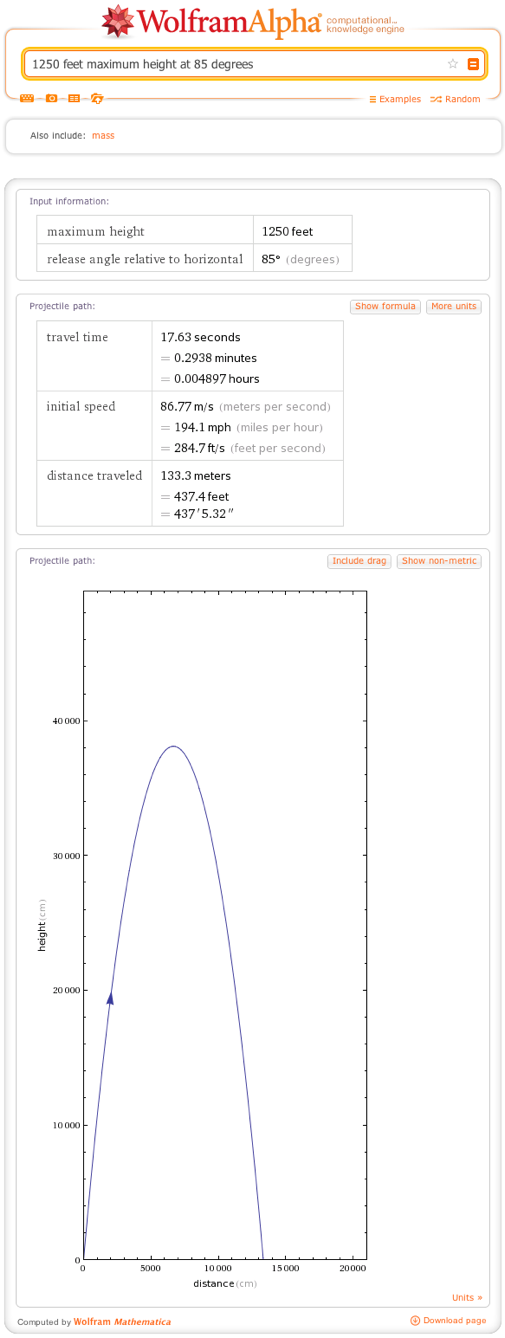Wolfram|Alpha includes tools for calculating more complicated forms of two-dimensional motion. Of particular note is a “mass on an inclined plane”. The force diagram is: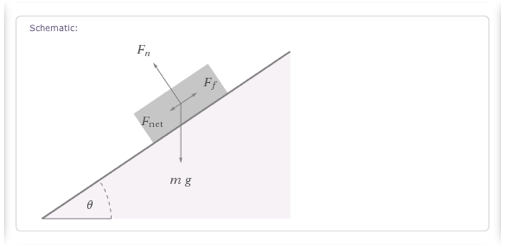Here we can see that the force of gravity is at an angle to the incline. That gives a component that is parallel to the surface of the incline and thus pulls the block downward and to the left. We also see a normal force indicated perpendicular to the surface of the slope. This is caused by Newton’s third law: that every action has an equal and opposite reaction. If we look at the force diagram of the sliding block, we can see that gravity is pushing down on the incline and thus the incline pushes back.

This normal force is important as a source of friction for objects sliding down an incline. Friction comes in two types for solid objects: static friction, where the two surfaces are at rest with respect to each other, and kinetic friction, for objects sliding past each other. You can include these forces in the Wolfram|Alpha formula, which allows you to model a block held in placed by the force of static friction or a block that is slowed by kinetic friction.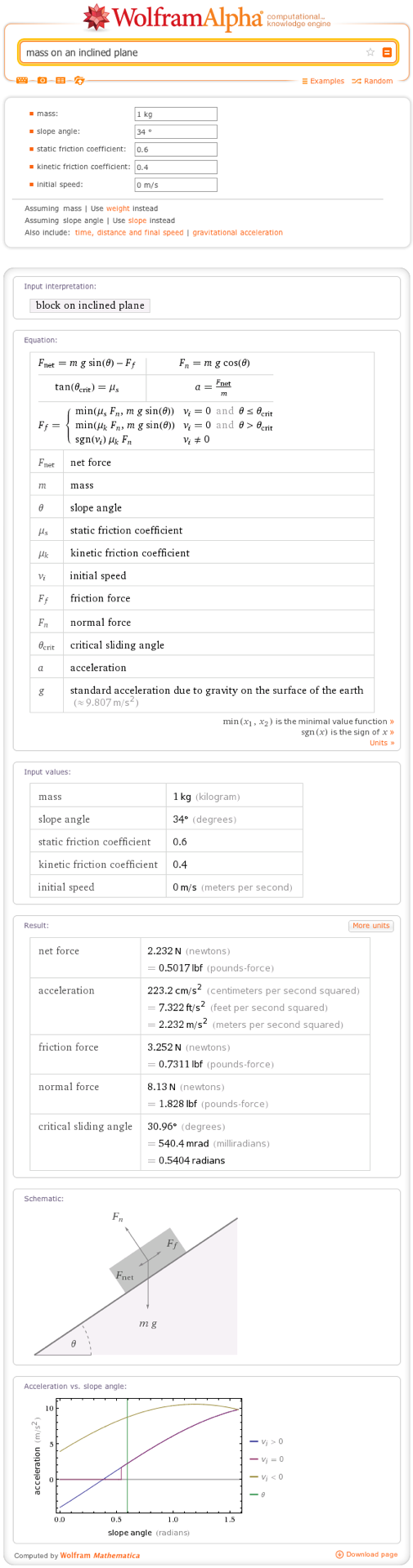All of this up and down motion brings up another key law of physics, conservation of energy. The law of conservation of energy states that energy can be destroyed or created. Energy can exist in many forms, but the primary division is between kinetic and potential energy. Objects in motion possess kinetic energy. For objects moving in a straight line, “kinetic energy” is defined as the work needed to accelerate an object from rest to its current velocity and is defined by the formula K = 1/2 mv2, where m is the mass of the object and v is its velocity.

What happens to the energy of an projectile when it is thrown into the air, like our superhero in the earlier example? We can see that the kinetic energy (and upward velocity) slowly declines as the projectile rises higher and then returns as it descends. But where does it go in between?

That is where the energy is converted into potential energy. This is the energy of an object due to its positioning, in this case its height above the Earth. This gravitation potential energy is roughly equal to the height above the Earth (subject to the small altitude limitation we discussed earlier). As the object rises into the air and then slows, its kinetic energy turns into potential energy until it finally falls back to the Earth and reverses the process.

For our superhero leaping a tall building in a single bound, if his initial velocity is 86.77 meters per second and he weighs about 70 kilograms, he would have an initial kinetic energy of 263.5 kilojoules. When he reached his maximum height just above the Empire State Building, he would have a gravitational potential energy of 261.5 kilojoules. The missing energy is his forward kinetic energy. This uses the horizontal part of his velocity, which is the “cosine of 85 degrees × 86.77 meters per second” or 7.563 meters per second, which gives a kinetic energy of 2.002 kilojoules. 261.5 kilojoules plus 2.002 kilojoules is equal to 263.5 kilojoules to our level of accuracy.

However, what about our block sliding down an incline plane with friction? It gains speed slower than it loses potential energy. Where does the energy go there? In that case, friction converts some of the energy of motion into heat, exciting the molecules and atoms that make up the surface of the incline and the sliding object. Heat is a kind of kinetic energy, where the vibrations and motion of the particles making up the plane and block increase. This is what causes the burns from a rope sliding through your hands or how rubbing your hands together in the cold can warm them faster than pressing them together.

Another important concept when it comes to energy is work. Work, as discussed for kinetic energy, can accelerate an object. It can also increase an object’s potential energy by moving it to a different position. But what is work? Work is the product of force and distance. Applying a force of 100 newtons to push a box 10 meters would yield 1 kilojoule, a unit of energy. Importantly, it is the product that matters. You can get the same amount of work done with less force if one increases the distance that force is applied. This idea underlies the mechanisms of many simple machines such as the “lever”.

Finally with energy, we also gain the concept of power, or the rate of energy transfer. Power is tied with how fast work is accomplished, and thus how quickly energy is added to a system. For example, the power needed to move a 100 kg object 10 meters in 5 seconds is 320 watts. But if we did it in 2 seconds, we would need 5 kilowatts. These measurements are more familiar to us from electromagnetism where they feature in the mechanics of “electric circuits”.

Newton’s third law of motion also implies the conservation of momentum. Force can be described in terms of momentum: F = p / t

Combining this with the idea of equal and opposite reactions, one can show that the sum of momenta of a system, not subject to outside forces, must remain constant. If two particles collide, their total momentum before the collision must be the same as after the collision.

Collisions are typically broken into two categories: elastic collisions, where both kinetic energy and momentum are conserved, and inelastic collisions, where the deformations of impact cause a loss of kinetic energy either by the particles sticking together or by some of the energy being converted into heat.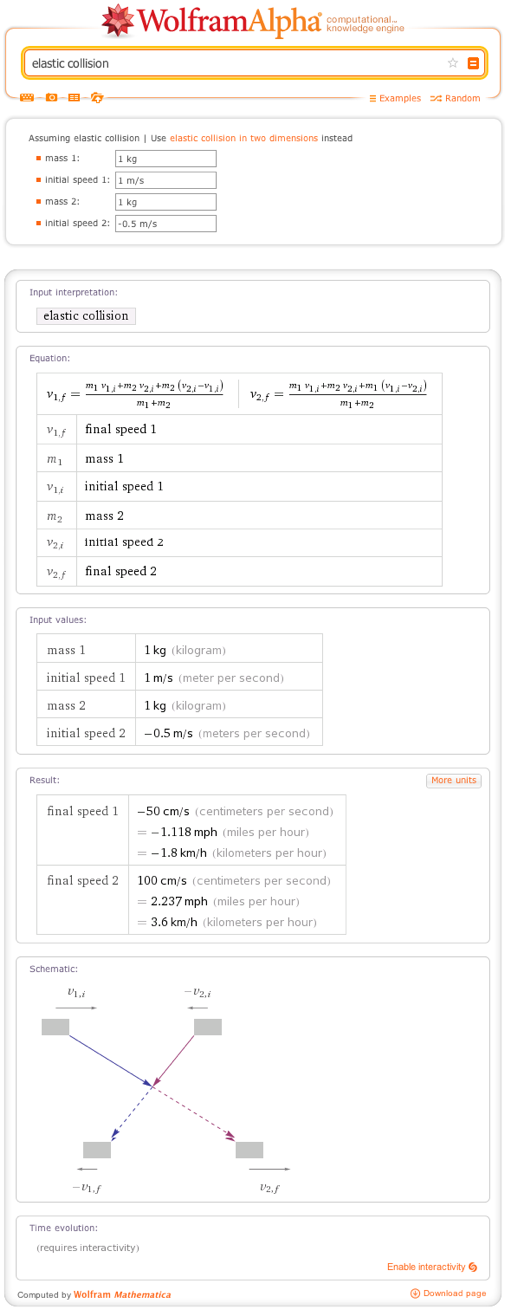The ideas here form the foundations of modern physics. Ideas about the conservation of energy and momentum go into Einstein’s famous equation E = m c2. The formulas for kinetic and potential energy tie into analytic mechanics and the formulations of the “Lagrangian” and “Hamiltonian” functions introduced in the eighteenth and nineteenth centuries. These in turn provided crucial concepts for the development of “quantum mechanics”. “Einstein’s theory of relativity” and the field of quantum mechanics in turn touch almost every other field in physics.

Thank you for the interesting article!

Posted by Matt May 9, 2013 at 3:28 pm

Hey Matt. What’s falling off of the Empire State building?

Posted by bsobddsaj November 19, 2013 at 4:06 pm

the content should be more clear

Posted by ANANYA ROY March 20, 2014 at 11:00 am

In my view this blog is extremely supportive in terms of theory and logical reasoning but when it comes to practical analysis and solving problems this blog does not provide such information

Posted by Radhika Jain June 6, 2014 at 11:30 pm

You are a very bright person!

Posted by Latesha Muske July 8, 2014 at 5:11 am

This Is So Intresting To Hear About Physics

Posted by Hezbon Maina August 16, 2014 at 10:32 am

I was never one for mathematics and physics but I suppose that having a basic understanding of how things moving around are affected by force and other factors can make it quite interesting when you look at every day things. But for the purposes of this article, let me just say that I think there are way too many theories being introduced here. I wanted to find out more about the law of motions but I think I’m never going to get the hang of it now!

Posted by Marcio Wilges February 4, 2015 at 10:50 pm

Physics has never been my favourite subject even since my childhood years. I find the numbers too daunting as they really confuse me though there are indeed many formulae for our easy reference. I am still able to recall a simple mathematical problem sum about a moving ball down the slope could take me more than an hour to solve. I guess I prefer simple math as compared to complicated theories that need time to decipher.

Posted by Audrey Greenwood February 8, 2015 at 10:57 pm

Thank you very much for taking the time to decipher a problem such as this. I’m a 67 year old grandmother and while Mathematica is not new to me WolframAlpha is, as well as all of the other fantastic sources available that I am now getting to know. I was a nontraditional physics major in that I started in my late 30s and 40s when my children were all in school. I became ill and could not get my degree. I’ve been disabled ever since and I can’t tell you what a blessing all of this is. (Mathematica, WolframAlpha, Math World – the whole shebang. I haven’t even scratched the surface of it all.) What a blessing all of this is to a reclusive, disabled older lady.

I just thought I’d give you a glimpse of a not so typical audience member (however, I’m sure I’m not alone) that thoroughly appreciated and enjoyed your work. Thanks!

Posted by Yvonne K Hunt August 11, 2015 at 4:09 am